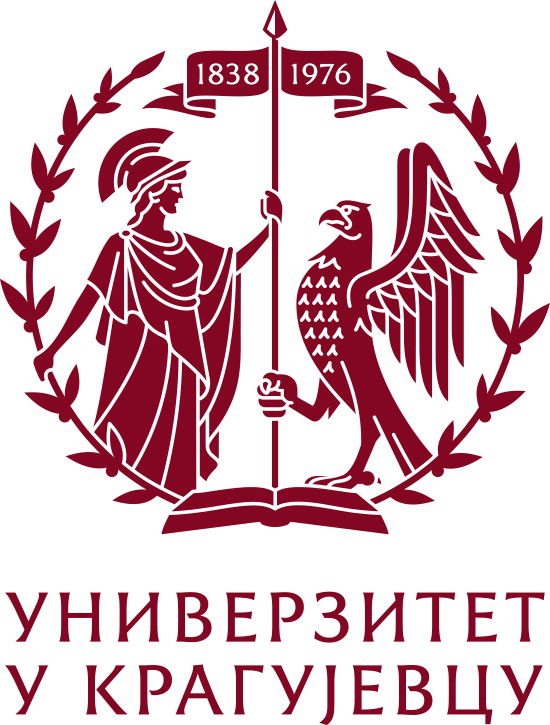Please use this identifier to cite or link to this item: `https://scidar.kg.ac.rs/handle/123456789/17453`
 Title: Kraus Operators for a Pair of Interacting Qubits: a Case Study Authors: Arsenijević, MomirIssue Date: 2017 Abstract: The Kraus form of the completely positive dynamical maps is appealing from the mathematical and the point of the diverse applications of the open quantum systems theory. The “integral”, i.e. so-called, Kraus form  of a com pletely positive dynamical map for an open quantum sys tem [2, 3] is appealing for the mathematical reasons. Mathematical existence of the Kraus form for such pro cesses is guaranteed by the Kraus theorem, universally [1-3]. On the other hand, a Kraus-form (KF) may be regarded as a solution to a differential master equation (ME) for the open system’s statistical operator (density matrix); a case when no ME exists for the process can be found e.g. in Refs. [4,5]. The Kraus operators are often constructed due to some physical assumptions or understanding of the underlying physical processes . Nevertheless, such derivations may not provide the full physical (e.g. microscopic) de tails . One way to obtain a proper KF for the open system’s dynamics is derivation from the related mas ter equation for the process [7,8]–if such an ME exists [4,5]. To this end, it is important to note: phenomeno logical derivations of MEs may also be unreliable–often there appear certain subtleties of both mathematical and physical nature as well as unexpected pitfalls [9,10]. Unfortunately, the Kraus operators are poorly known for the two-qubit processes. Having this in mind as well as the above-distinguished usefulness of KF, in this pa per we derive the Kraus operators starting from a microscopically derived master equation for a pair of twolevel systems (qubits). We are concerned with an ancilla qubit interacting with another qubit, which is subjected to a Sx spin-projection quantum measurement, while the strength of the interaction is arbitrary. Usefulness of the KF for the process is emphasized by application of our results in investigating the dynamics of entanglement in the qubits system. The obtained results are applied to calculate the dynamics of the initial entanglement in the qubits system. We obtain the loss of the correlations in the finite time interval; the stronger the inter-qubit interaction, the longer lasting entanglement in the system. URI: https://scidar.kg.ac.rs/handle/123456789/17453 Type: conferenceObject Appears in Collections: Faculty of Science, Kragujevac35